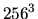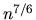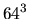The case for scalable linear solvers

Computer simulations play an increasingly important role in scientific and engineering investigations, supplementing (and in some cases, replacing) traditional experiments. In engineering applications, such as aircraft design or crash studies, numerical simulation is far cheaper than experimentation. In other applications, such as weather modelling or the study of climate change, experiments are impractical. The importance of computer simulation is highlighted by the ambitious ASCI project of the USA where, in the area of nuclear weapons testing and stockpile stewardship, full-blown experiments are prohibited by the Comprehensive Test Ban Treaty, and detailed and accurate numerical simulations are needed as an alternative. Towards this end, high fidelity codes are being developed to solve three-dimensional problems that require the computational speed and large memory of the massively parallel computers developed for the ASCI programme.

High performance computers alone are however not sufficient for the solution of such problems. Scalable numerical algorithms that can exploit such high performance machines of today and the future are equally essential. Many of the algorithms used in today's simulation codes are based on yesterday's unscalable technology. This means that the work required to solve increasingly large problems grows much faster than linearly (the optimal rate). The use of scalable algorithms could decrease simulation times by orders of magnitude, and therefore enable application scientists and engineers to do larger simulations at higher resolutions than would not otherwise be permissible using conventional algorithms.

In many large-scale scientific and industrial simulation codes, the majority of the run time is spent in a linear solver. For this reason, research and development of scalable solvers for these large, sparse linear systems of equations on parallel computers is of vital importance.

SAM: a scalable algebraic multigrid solver

The multigrid method is an example of a scalable linear solver. It uses a ``smoother'', for example, the Gauss-Seidel algorithm, to damp efficiently high-frequency errors, leaving only low-frequency, or smooth, errors. The low-frequency errors can be accurately and efficiently solved for on a coarser (smaller) grid. Recursive application of the procedure leads to the multigrid algorithm. This algorithm uniformly damps all error frequencies with a computational cost that depends only linearly on the problem size. In other words, multigrid algorithms are scalable.

There are two basic multigrid approaches: geometric and algebraic. In geometric multigrid, the geometry of the problem is used to define the various multigrid components. In contrast, algebraic multigrid methods use only the information available from the matrix of the linear system of equations.

For linear systems defined on structured meshes, we have developed a parallel geometric multigrid algorithm. This algorithm was used in the three-dimensional parallel DNS simulation of combustion (using a mesh ofcells)  on the UK's (then) most powerful machine - the Cray T3D at the University of Edinburgh. It converged twice as quickly in CPU time when compared with a conjugate gradient algorithm with MILU preconditioner on 256 processors.

For linear systems defined on unstructured meshes, it is difficult to develop geometric multigrid algorithms that are simple and portable from application to application. For this reason, we wish to develop new algebraic multigrid (AMG) algorithms. This type of algorithms was first proposed in [4,8]. AMG constructs a hierarchy of coarser levels in an automatic way without the explicit use of the mesh information. All of the information required for this setup is taken from the given matrix. Over the years a number of alternative approaches towards algebraic multigrid have been suggested. These include ILU or block elimination based approaches [1,2,3], as well as aggregation or agglomeration type methods [9,10].

However the use of Krylov subspace algorithms has dominated over the use of AMG for a long period until recently, when the potential of AMG has been recognised, leading to an increasing interest. This renewed interest has arisen from two reasons. First of all, AMG has demonstrated an ability to solve difficult problems related to irregular meshes of high aspect ratio and/or anisotropic problems, on which ILU based Krylov subspace algorithms have difficulties. Secondly, the size of the systems to be solved has increased with demand for high fidelity models for simulation. Theoretically AMG, as a multigrid algorithm, has a complexity linear in the size of the problem. In other words the number of iterations for AMG to converge is independent of the problem size. This is in contrast to ILU based Krylov subspace algorithms, where the complexity is approximate O() and therefore the number of iterations to convergence increases with the problem size. For instance, in our previous study , an MILU conjugate gradient algorithm took 144 iterations to solve a system of sizeon 16 processors. The same algorithm took 474 iterations to solve the linear system of sizeon 256 processors.

There are however difficulties with AMG. These include the robustness of AMG for non-L-type matrices and the relatively high memory requirement of AMG. So far, there has been only limited success in solving these problems. In , operator-dependent interpolation based on Jacobi iteration was proposed to improve the robustness of AMG and thus allow more aggressive coarsening. In , modification to the interpolation formula of  was suggested to deal with systems having positive off-diagonal elements. Parallelisation of AMG is another important issue. Unlike Krylov methods such as conjugate gradients, AMG is far more difficult to parallelise. These problems need to be addressed before AMG can be widely adopted as the preferred scalable solver for large scale applications. The software SAM is an effort towards this direction.

2000-03-22